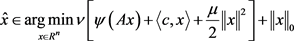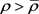# 一类零模正则复合优化问题的多阶段凸松弛法GEP-MSCRA for a Kind of Zero-Norm Regularized Composite Optimization Problem

DOI: 10.12677/ORF.2019.91008, PDF, HTML, XML, 下载: 466  浏览: 710

Abstract: This article starts from the variational characterization of zero-norm, then changes such a combi-nation optimization problem to an equivalent model which has bi-linear structure and global Lip-schitz continuous. This article also designed multi-stage convex relaxation methods to solve the zero-norm regularized composite optimization problem, and analyzed the convergence for it.

1. 引言

2. 零模正则复合优化问题

2.1. 零模正则问题

${R}^{n}$ 是赋予了内积 $〈\cdot ,\cdot 〉$ 及诱导范数 $‖\cdot ‖$ 的有限维向量空间。考虑零模正则规划：(1)

2.2. 零模问题的等价Lipschitz替代

$\stackrel{¯}{t}$${\left(\partial \varphi \right)}^{-1}\left(\frac{1}{1-{t}^{\ast }}\right)\cap \left[{t}^{\ast },1\right)$ 中的最小元素，由下面引理(参考文献  引理5)可知这样的 $\stackrel{¯}{t}$ 存在：

$\left\{\begin{array}{cc}\begin{array}{l}{v}^{\ast }=1,\\ {v}^{\ast }\ge \frac{\rho \omega \left(1-{t}_{0}\right)}{{{\varphi }^{\prime }}_{-}\left(1\right)\left(1-{t}^{\ast }\right)},\\ {v}^{\ast }\ge \rho \omega \left(1-{t}_{0}\right),\end{array}& \begin{array}{l}若\rho \omega \in \left({{\varphi }^{\prime }}_{-}\left(1\right),+\infty \right);\\ 若\rho \omega \in \left[\frac{1}{1-{t}^{\ast }},{{\varphi }^{\prime }}_{-}\left(1\right)\right];\\ 若\rho \omega \in \left[0,\frac{1}{1-{t}^{\ast }}\right).\end{array}\end{array}$

${‖x‖}_{0}=\underset{\theta \in {R}^{n}}{\mathrm{min}}\left\{\underset{i=1}{\overset{n}{\sum }}\varphi \left({\theta }_{i}\right):{‖x‖}_{1}-〈\theta ,|x|〉=0,0\le \theta \le e\right\}$ (2)

$\underset{x\in {R}^{n},\theta \in {R}^{n}}{\mathrm{min}}\left\{\nu f\left(x\right)+\underset{i=1}{\overset{n}{\sum }}\varphi \left({\theta }_{i}\right):〈e-\theta ,|x|〉=0,0\le \theta \le e\right\}$ (3)

$\underset{x\in {R}^{n},\theta \in \left[0,e\right]}{\mathrm{min}}\left\{vf\left(x\right)+\underset{i=1}{\overset{n}{\sum }}\left[\varphi \left({\theta }_{i}\right)+\rho \left(1-{\theta }_{i}\right)|{x}_{i}|\right]\right\}$ (4)

$\left(\stackrel{^}{x},\stackrel{^}{\theta }\right)\in \underset{x\in {R}^{n},\theta \in \left[0,e\right]}{\mathrm{min}}\left\{vf\left(x\right)+\underset{i=1}{\overset{n}{\sum }}\left[\varphi \left({\theta }_{i}\right)+\rho \left(1-{\theta }_{i}\right)|{x}_{i}|\right]\right\}$ ，其中 $\rho >\stackrel{¯}{\rho }$(5)

$\underset{x\in {R}^{n},\theta \in {R}^{n}}{\mathrm{min}}\left\{vf\left(x\right)+\rho {‖x‖}_{1}+\underset{i=1}{\overset{n}{\sum }}\left[\varphi \left({\theta }_{i}\right)-\rho {\theta }_{i}|{x}_{i}|\right]\right\}$

$\stackrel{^}{x}\in \underset{x\in {R}^{n}}{\mathrm{arg}\mathrm{min}}\left\{vf\left(x\right)+\rho {‖x‖}_{\text{1}}-\underset{\text{i}=\text{1}}{\overset{\text{n}}{\sum }}{\phi }^{\ast }\left(\rho |{x}_{i}|\right)\right\}$ ，其中 $\rho >\stackrel{¯}{\rho }$ (6)

3. 多阶段凸松弛法

Step 0：取 $\varphi \in \Phi$$v>0$ 和初始点 ${\theta }^{0}\in \left[0,\stackrel{¯}{t}e\right]$。置 $k:=1$

Step 1：令 ${v}^{k-1}=e-{\theta }^{k-1}$ 并计算下面的极小化问题： ${x}^{k}\in \underset{x\in {R}^{n}}{\mathrm{arg}\mathrm{min}}\left\{f\left(x\right)+\lambda {\sum }_{i=1}^{n}{v}_{i}^{k-1}|{x}_{i}|\right\}$(7)

$k=1$ ，由 ${x}^{1}$ 的取值选取参数并使 $\lambda =\rho {v}^{-1}$

Step 2：计算下面的极小化问题： ${\theta }^{k}\in \underset{\theta \in \left[0,e\right]}{\mathrm{arg}\mathrm{min}}\left\{{\sum }_{i=1}^{n}\left[\varphi \left({\theta }_{i}\right)-\rho {\theta }_{i}|{x}_{i}^{k}|\right]\right\}$(8)

Step 3：置 $k←k+1$ ，返回(S. 1)步。

4. 理论分析

$C\left(\stackrel{¯}{S},l\right):=\left\{x\in {R}^{n}:\exists S\supseteq \stackrel{¯}{S}且|S|\le l,使得{\sum }_{i\in {S}^{c}}|{x}_{i}|\le \frac{2}{1-\stackrel{¯}{t}}{\sum }_{i\in S}|{x}_{i}|\right\}$

$E\left[\mathrm{exp}\left(t{\epsilon }_{i}\right)\right]\le \mathrm{exp}\left(\frac{{\sigma }^{2}{t}^{2}}{2}\right)$

$\psi \left(A{x}^{k}\right)+〈c,{x}^{k}〉+\frac{\mu }{2}{‖{x}^{k}‖}^{2}+\lambda {\sum }_{i=1}^{n}{v}_{i}^{k-1}|{x}_{i}^{k}|\le \psi \left(A\stackrel{¯}{x}\right)+〈c,\stackrel{¯}{x}〉+\frac{\mu }{2}{‖\stackrel{¯}{x}‖}^{2}+\lambda {\sum }_{i=1}^{n}{v}_{i}^{k-1}|{\stackrel{¯}{x}}_{i}|$

$\begin{array}{l}\psi \left(A{x}^{k}\right)-\psi \left(A\stackrel{¯}{x}\right)+〈c,{\Delta }^{k}〉+\frac{\mu }{2}{\left(‖{x}^{k}‖}^{2}-{‖\stackrel{¯}{x}‖}^{2}\right)-〈\stackrel{^}{\epsilon },{\Delta }^{k}〉\\ \text{ }\text{ }\text{ }\le -〈\stackrel{^}{\epsilon },{\Delta }^{k}〉+\lambda {\sum }_{i=1}^{n}{v}_{i}^{k-1}\left(|{\stackrel{¯}{x}}_{i}|-|{x}_{i}^{k}|\right)\\ \text{ }\text{ }\text{ }\le {\sum }_{i=1}^{n}|{\stackrel{^}{\epsilon }}_{i}||{\Delta }_{i}^{k}|+\lambda {\sum }_{i\in \stackrel{¯}{S}}{v}_{i}^{k-1}\left(|{\stackrel{¯}{x}}_{i}|-|{x}_{i}^{k}|\right)-\lambda {\sum }_{i\in {\stackrel{¯}{S}}^{c}}{v}_{i}^{k-1}|{x}_{i}^{k}|\\ \text{ }\text{ }\text{ }\le {\sum }_{i=1}^{n}|{\stackrel{^}{\epsilon }}_{i}||{\Delta }_{i}^{k}|+\lambda {\sum }_{i\in \stackrel{¯}{S}}{v}_{i}^{k-1}|{\Delta }_{i}^{k}|-\lambda {\sum }_{i\in {\left({S}^{k-1}\right)}^{c}}{v}_{i}^{k-1}|{\Delta }_{i}^{k}|\\ \text{ }\text{ }\text{ }\le {\sum }_{i\in {S}^{k-1}\\stackrel{¯}{S}}|{\stackrel{^}{\epsilon }}_{i}||{\Delta }_{i}^{k}|+\left(\lambda +{‖\stackrel{^}{\epsilon }‖}_{\infty }\right){\sum }_{i\in \stackrel{¯}{S}}|{\Delta }_{i}^{k}|+{\left(‖\stackrel{^}{\epsilon }‖}_{\infty }-\lambda \left(1-\stackrel{¯}{t}\right)\right){\sum }_{i\in {\left({S}^{k-1}\right)}^{c}}|{\Delta }_{i}^{k}|\end{array}$ (9)

$\left(\lambda \left(1-\stackrel{¯}{t}\right)-{‖\stackrel{^}{\epsilon }‖}_{\infty }\right){\sum }_{i\in {\left({S}^{k-1}\right)}^{c}}|{\Delta }_{i}^{k}|\le {\sum }_{i\in {S}^{k-1}\\stackrel{¯}{S}}|{\stackrel{^}{\epsilon }}_{i}||{\Delta }_{i}^{k}|+\left(\lambda +{‖\stackrel{^}{\epsilon }‖}_{\infty }\right){\sum }_{i\in \stackrel{¯}{S}}|{\Delta }_{i}^{k}|\le \left(\lambda +{‖\stackrel{^}{\epsilon }‖}_{\infty }\right){\sum }_{i\in {S}^{k-1}}|{\Delta }_{i}^{k}|$ (10)

$‖{\Delta }^{k}‖\le \frac{1}{{\gamma }_{k}}\left(‖{\stackrel{^}{\epsilon }}_{{S}^{k-1}}‖+\lambda \sqrt{{\sum }_{i\in \stackrel{¯}{S}}{\left({v}_{i}^{k-1}\right)}^{2}}\right)$

$\begin{array}{c}{\gamma }_{k}{‖{\Delta }^{k}‖}^{2}\le \left({\gamma }_{k}+\mu /2\right){‖{\Delta }^{k}‖}^{2}\le {\sum }_{i=1}^{n}|{\stackrel{^}{\epsilon }}_{i}||{\Delta }_{i}^{k}|+\lambda {\sum }_{i\in \stackrel{¯}{S}}{v}_{i}^{k-1}|{\Delta }_{i}^{k}|-\lambda {\sum }_{i\in {\left({S}^{k-1}\right)}^{c}}{v}_{i}^{k-1}|{\Delta }_{i}^{k}|\\ \le {\sum }_{i=1}^{n}|{\stackrel{^}{\epsilon }}_{i}||{\Delta }_{i}^{k}|+\lambda {\sum }_{i\in \stackrel{¯}{S}}{v}_{i}^{k-1}|{\Delta }_{i}^{k}|-\lambda \left(1-\stackrel{¯}{t}\right){\sum }_{i\in {\left({S}^{k-1}\right)}^{c}}|{\Delta }_{i}^{k}|\\ \le {\sum }_{i\in {S}^{k-1}}|{\stackrel{^}{\epsilon }}_{i}||{\Delta }_{i}^{k}|+\lambda {\sum }_{i\in \stackrel{¯}{S}}{v}_{i}^{k-1}|{\Delta }_{i}^{k}|\\ \le \sqrt{{\sum }_{i\in {S}^{k-1}}{|{\stackrel{^}{\epsilon }}_{i}|}^{2}}‖{\Delta }^{k}‖+\lambda \sqrt{{\sum }_{i\in \stackrel{¯}{S}}{\left({v}_{i}^{k-1}\right)}^{2}}‖{\Delta }^{k}‖\end{array}$ (11)

$‖{x}^{k}-\stackrel{¯}{x}‖\le \left\{\begin{array}{c}\frac{{v}^{-1}\left(4-2\stackrel{¯}{t}\right)}{\kappa \left(3-\stackrel{¯}{t}\right)}\sqrt{1.5\stackrel{¯}{r}},若k=1;\\ \frac{\rho {v}^{-1}\left(4-2\stackrel{¯}{t}\right)}{\kappa \left(3-\stackrel{¯}{t}\right)}\sqrt{1.5\stackrel{¯}{r}},若k=2,3,\cdots .\end{array}$

$‖{x}^{k}-\stackrel{¯}{x}‖\le \frac{\sqrt{3}}{\kappa \left(\sqrt{3}-1\right)}\left[‖{\stackrel{^}{\epsilon }}_{\stackrel{¯}{S}}‖+\rho {v}^{-1}\sqrt{{\sum }_{i\in \stackrel{¯}{S}}{Ι}_{\Lambda }\left(i\right)}\right]+{\left(\frac{1}{\sqrt{3}}\right)}^{k-1}‖{x}^{1}-\stackrel{¯}{x}‖$

5. 结论

  Loh, P.L. and Wainwright, M.J. (2015) Regularized M-Estimators with Nonconvexity: Statistical and Algorithmic Theory for Local Optima. Journal of Machine Learning Research, 16, 559-616.  Casasaya, J. and Llibre, J. (2009) Large-Scale Sparse Logistic Regression. ACM Press, New York, 547-556.  Rockafellar, R.T. (1970) Convex Analysis. Princeton University Press.  Bi, S.J., Liu, X.L. and Pan, S.H. (2014) Exact Penalty Decomposition Method for Zero-Norm Minimization Based on MPEC Formulation. SIAM Journal on Scientific Computing, 36, 1451-1477. https://doi.org/10.1137/110855867  贲树军. 低秩优化问题的多阶段凸松弛法研究[D]: [博士学位论文]. 广州: 华南理工大学, 2014.  Candes, E.J., Wakin, M.B. and Boyd, S.P. (2008) Enhancing Sparsity by Reweighted l1 Minimization. Journal of Fourier Analysis and Applications, 14, 877-905. https://doi.org/10.1007/s00041-008-9045-x  Schmidt, M., Fung, G. and Rosales, R. (2007) Fast Optimization Methods for l1 Regularization: A Comparative Study and Two New Approaches. Lnai, 4701, 286-297. https://doi.org/10.1007/978-3-540-74958-5_28  Park, M.Y. and Hastie, T. (2008) L1 Regularized Path Algorithm for Gen-eralized Linear Models. Journal of the Royal Statistical Society: Series B, 69, 659-677. https://doi.org/10.1111/j.1467-9868.2007.00607.x  Koh, K., Kim, S. and Boyd, S. (2007) An Interior-Point Method for Large-Scale l1-Regularized Logistic Regression. Journal of Machine Learning Research, 8, 1519-1555.  Lee, S., Lee, H., Abbeel, P. and Ng, A.Y. (2006) Efficient l1 Regularized Logistic Regression. National Conference on Artificial Intelligence, 1, 401-408.  Loh, P.L. and Wainwright, M.J. (2015) Regularized M-Estimators with Nonconvexity: Statistical and Algorithmic Theory for Local Optima. Journal of Machine Learning Research, 16, 559-616.  Casasaya, J. and Llibre, J. (2009) Large-Scale Sparse Logistic Regression. ACM Press, New York, 547-556.  Rockafellar, R.T. (1970) Convex Analysis. Princeton University Press.  Bi, S.J. and Pan, S.H. (2017) GEP-MSCRA for Computing the Group Ze-ro-Norm Regularized Least Squares Estimator. arXiv.org.  贲树军. 低秩优化问题的多阶段凸松弛法研究[D]: [博士学位论文]. 广州: 华南理工大学, 2014.  Candes, E.J., Wakin, M.B. and Boyd, S.P. (2008) Enhancing Sparsity by Reweighted l1 Minimization. Journal of Fourier Analysis and Applications, 14, 877-905. https://doi.org/10.1007/s00041-008-9045-x  Schmidt, M., Fung, G. and Rosales, R. (2007) Fast Optimization Methods for l1 Regularization: A Comparative Study and Two New Approaches. Lnai, 4701, 286-297. https://doi.org/10.1007/978-3-540-74958-5_28  Park, M.Y. and Hastie, T. (2008) L1 Regularized Path Algorithm for Generalized Linear Models. Journal of the Royal Statistical Society: Series B, 69, 659-677. https://doi.org/10.1111/j.1467-9868.2007.00607.x  Koh, K., Kim, S. and Boyd, S. (2007) An Interior-Point Method for Large-Scale l1-Regularized Logistic Regression. Journal of Machine Learning Research, 8, 1519-1555.  Lee, S., Lee, H., Abbeel, P. and Ng, A.Y. (2006) Efficient l1 Regularized Logistic Regression. National Conference on Artificial Intelligence, 1, 401-408.